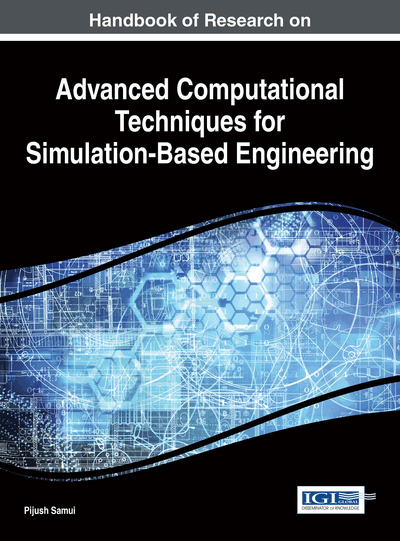# Multiobjective Optimization in Water and Environmental Systems Management- MODE Approach

Janga Reddy Manne (Indian Institute of Technology, India)
DOI: 10.4018/978-1-4666-9479-8.ch004

## Abstract

Many real world problems are characterized by multiple goals, often conflicting in nature and compete with one another. Multi-objective optimization problems (MOOPs) require the simultaneous optimization of several non-commensurable and conflicting objectives. In the past, several studies have used conventional approaches to solve the MOOPs by adopting weighted approach or constrained approach, which may face difficulties while generating Pareto optimal solutions, if optimal solution lies on non-convex or disconnected regions of the objective function space. An effective algorithm should have an ability to learn from earlier performance to direct proper selection of weights for further evolutions. To achieve these goals, multi-objective evolutionary algorithms (MOEAs) have become effective means in recent past, which can generate a population of solutions in each iteration and offer a set of alternatives in a single run. This chapter presents an effective MOEA, namely multi-objective differential evolution (MODE) for problems of solving water, environmental systems.
Chapter Preview
Top

## Introduction

Many times, most of the problems in water and environmental systems are characterized by multiple goals, which are often conflicting in nature and compete with one another. These multi-objective optimization problems (MOOPs) require the simultaneous optimization of several non-commensurable and conflicting objectives. Because of the contradictory objectives, it is not possible to find a single optimal solution, which will satisfy all the goals. Instead, the solutions exists in the form of alternative trade-offs, also known as the Pareto optimal solutions.

In the past, several studies have used conventional optimization techniques to solve the multi-objective problems by adopting weighted sum approach or constrained optimization approach. The conventional approaches may face difficulties while generating optimal solutions for practical problems, if optimal solution lies on non-convex or disconnected regions of the objective function space. While using classical optimization methods such as linear programming (LP), dynamic programming (DP) and non-linear programming (NLP) to solve the multi-objective problems, they may face difficulties in generating Pareto optimal solutions (Reddy and Kumar 2006). In the weighted sum method, the multiple objectives of the problem will be converted into a single objective optimization by adopting suitable weights to objectives. By using a single pair of fixed weights, only one point on Pareto front can be obtained. Therefore, if one would like to obtain the complete Pareto front, all possible Pareto solutions must first be derived. This requires the algorithm to be executed iteratively, so as to guarantee that every weight combination has been evaluated. Obviously, it is impractical to reiterate the algorithm continually to exhaust all the weight combinations. Similarly, in the constraint method, it needs to reiterate the algorithm for a large number of times, which requires more computational effort. Also conventional methods may face problems, if optimal solution lies on non-convex or disconnected regions of the objective function space. Thus the classical methods are not ideal approaches to solve multi-objective optimization problems (MOOP).

In the process of developing a multi-objective algorithm for solution of an MOOP, it should have an ability to “learn” from previous performance to direct proper selection of weights for further evolutions. To achieve these goals, multi-objective evolutionary algorithms (MOEAs) have become effective means in recent past (Deb, 2002). Due to their efficiency and easiness to handle non-linear relationships, ability to approximate the non-convex and disconnected Pareto optimal fronts of real-world problems, MOEAs are getting diverse applications in various fields. Apart from that, the specific advantage of MOEAs over the classical optimization techniques is that they generate a population of solutions in each iteration and offer a set of alternatives (Pareto optimal set) in a single run. Thus MOEAs being population based stochastic search techniques are becoming popular to solve multi-objective engineering problems. This chapter proposes an effective multi-objective optimization algorithm, namely multi-objective differential evolution (MODE) for generating Pareto optimal solutions in water and environmental systems. In the following sections, first the basic principles and issues in developing MOEAs are discussed. Subsequently, brief details of past works on differential evolution for multi-objective optimization are reviewed, and an effective multi-objective procedure namely Multi-Objective Differential Evolution (MODE) is presented. Afterward, the performance of MODE was tested for few numerical problems, and demonstrated the application of MODE for reservoir operation problem. Finally, a brief discussion on future research directions and conclusions are presented.

## Key Terms in this Chapter

Crowding Distance: The crowding distance value of a solution provides an estimate of the density of solutions surrounding that solution. The crowding distance value of a particular solution is the average distance of its two neighboring solutions.

Multi-Objective Optimization: It deals with decision making for problems that require simultaneous optimization of several non-commensurable and often competitive/conflicting objectives.

Pareto Optimal Solution: The Pareto Optimal Solution refers to a solution, around which there is no way of improving any objective without degrading at least one other objective.

Pareto Front: Pareto Front is a set of nondominated solutions, being chosen as optimal, if no objective can be improved without sacrificing at least one other objective. On the other hand a solution x* is referred to as dominated by another solution x if, and only if, x is equally good or better than x* with respect to all objectives.

Set Coverage Metric: It gives the relative spread of solutions between two sets of solution vectors.

DE Algorithm: Differential evolution (DE) is a method that optimizes a problem by iteratively trying to improve a candidate solution with regard to a given measure of quality.

Spacing Metric: It evaluates the spread (distribution) of vectors throughout the set of non-dominated solutions, and is calculated with a relative distance measure between consecutive solutions in the obtained non-dominated set.

## Complete Chapter List

Search this Book:
Reset